Theory and Modern Applications

# Some results of certain types of difference and differential equations

## Abstract

In this article, we shall utilize the value distribution theory and complex oscillation theory to investigate certain types of difference and differential equations. The results we obtain generalize some previous results of Gundersen and Yang.

MSC:30D35, 34M10.

## 1 Introduction and main results

In this paper, we assume that the reader is familiar with the fundamental results and the standard notations of the Nevanlinna theory (e.g., see ). In addition, we will use the notation $\sigma \left(f\right)$ to denote the order of the meromorphic function $f\left(z\right)$. We recall the definition of hyper-order (see ), ${\sigma }_{2}\left(f\right)$ of $f\left(z\right)$ is defined by

${\sigma }_{2}\left(f\right)=\underset{r\to \mathrm{\infty }}{lim sup}\frac{loglogT\left(r,f\right)}{logr}.$

Let f and g be two non-constant meromorphic functions in the complex plane. By $S\left(r,f\right)$, we denote any quantity satisfying $S\left(r,f\right)=o\left(T\left(r,f\right)\right)$ as $r\to \mathrm{\infty }$, possibly outside a set of r with finite linear measure. Then the meromorphic function β is called a small function of f, if $T\left(r,\beta \right)=S\left(r,f\right)$. If $f-\beta$ and $g-\beta$ have the same zeros, counting multiplicity (ignoring multiplicity), then we say f and g share the small function β CM (IM).

Let ${z}_{0}$ be a zero of $f-\beta$ with multiplicity p and a zero of $g-\beta$ with multiplicity q. We denote by ${N}_{L}\left(r,\frac{1}{f-\beta }\right)$ the counting function of the zeros of $f-\beta$ where $p>q\ge 1$, each point counted $p-q$ times. In the same way, we also define ${N}_{L}\left(r,\frac{1}{g-\beta }\right)$.

Let $f\left(z\right)$ be transcendental meromorphic function in the plane, $c\in \mathbb{C}\setminus \left\{0\right\}$ be a constant such that $f\left(z\right)\not\equiv f\left(z+c\right)$. The forward differences ${\mathrm{\Delta }}^{n}f\left(z\right)$ are defined in the standard way  by

$\mathrm{\Delta }f\left(z\right)=f\left(z+c\right)-f\left(z\right),\phantom{\rule{2em}{0ex}}{\mathrm{\Delta }}^{n+1}f\left(z\right)={\mathrm{\Delta }}^{n}f\left(z+c\right)-{\mathrm{\Delta }}^{n}f\left(z\right),\phantom{\rule{1em}{0ex}}n=0,1,\dots .$

In 1996, Brück raised the following conjecture:

Conjecture Let f be a non-constant entire function such that the hyper order ${\sigma }_{2}\left(f\right)<\mathrm{\infty }$ and ${\sigma }_{2}\left(f\right)$ is not a positive integer. If f and ${f}^{\prime }$ share the finite value a CM, then

$\frac{{f}^{\prime }-a}{f-a}=c,$

where c is a nonzero constant.

The case that $a=0$ had been proved by Brück himself in . From differential equations

$\frac{{f}^{\prime }-1}{f-1}={e}^{{z}^{n}},\phantom{\rule{2em}{0ex}}\frac{{f}^{\prime }-1}{f-1}={e}^{{e}^{z}},$

we see that when the hyper order ${\sigma }_{2}\left(f\right)$ of f is a positive integer or infinite, the conjecture of Brück does not hold.

Gundersen and Yang proved the conjecture holds for entire functions of finite order, see , and Yang generalized this finite order for ${f}^{\left(k\right)}$ ($k\ge 1$), instead of ${f}^{\prime }$, see . Chen and Shon proved the conjecture holds if ${\sigma }_{2}\left(f\right)<\frac{1}{2}$, see . In terms of sharing a small function α IM, recently Wang has generalized Gundersen and Yang’s results, see .

In this paper, we consider the uniqueness of entire functions sharing a small function with their linear difference and differential polynomial. Now we present the main theorems.

Theorem 1.1 Let $f\left(z\right)$ be a non-constant entire function, ${\sigma }_{2}\left(f\right)$ (<∞) is not a positive integer. Set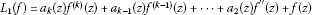($k\ge 2$), where ${a}_{j}\left(z\right)$ ($2\le j\le k$) are entire functions of order less than 1 and ${a}_{k}\left(z\right)\not\equiv 0$. If $f\left(z\right)$ and ${L}_{1}\left(f\right)$ share z IM, and

$s:=max\left\{\underset{r\to \mathrm{\infty }}{lim sup}\frac{log\left({N}_{L}\left(r,\frac{1}{f\left(z\right)-z}\right)\right)}{logr},\underset{r\to \mathrm{\infty }}{lim sup}\frac{log\left({N}_{L}\left(r,\frac{1}{{L}_{1}\left(f\right)-z}\right)\right)}{logr}\right\}<1,$
(1.1)

then ${L}_{1}\left(f\right)-z=h\left(z\right)\left(f\left(z\right)-z\right)$, where $h\left(z\right)$ is a meromorphic function of order no greater than s.

Remark 1 Note that the term ${f}^{\prime }\left(z\right)$ cannot be contained in ${L}_{1}\left(f\right)$, otherwise Theorem 1.1 does not hold. For example: Set $f\left(z\right)=2{e}^{-z}+z$ and ${L}_{1}\left(f\right)={f}^{″}\left(z\right)+2{f}^{\prime }\left(z\right)+f\left(z\right)$. Then $f\left(z\right)$ and ${L}_{1}\left(f\right)$ share z IM, but

$\frac{{L}_{1}\left(f\right)-z}{f\left(z\right)-z}={e}^{z}.$

Theorem 1.2 Let $f\left(z\right)$ be a non-constant entire function, ${\sigma }_{2}\left(f\right)$ (<∞) is not a positive integer. Set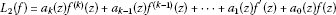($k\ge 1$), where ${a}_{j}\left(z\right)$ ($0\le j\le k$) are entire functions and $max\left\{\sigma \left({a}_{j}\right)|j=1,2,\dots ,k\right\}<\sigma \left({a}_{0}\right)\in \mathbb{N}$. If $f\left(z\right)$ and ${L}_{2}\left(f\right)$ share a IM (a is a constant), then

${L}_{2}\left(f\right)-a=h\left(z\right)\left(f\left(z\right)-a\right),$

where $h\left(z\right)$ is a meromorphic function of order no less than 1.

Theorem 1.3 Let $f\left(z\right)$ be a non-constant entire function of order less than $\frac{1}{2}$ and $a\left(z\right)$ be a non-zero small function of $f\left(z\right)$. Set $A\left(f\right)={a}_{k}\left(z\right){\mathrm{\Delta }}^{k}f\left(z\right)+\cdots +{a}_{1}\left(z\right)\mathrm{\Delta }f\left(z\right)+{a}_{0}\left(z\right)f\left(z\right)$, where ${a}_{j}\left(z\right)$ ($j=0,1,\dots ,k$) are polynomial and ${a}_{k}\left(z\right)\not\equiv 0$. If $f\left(z\right)-a\left(z\right)=0\to A\left(f\right)-a\left(z\right)=0$, then

$\frac{A\left(f\right)-a\left(z\right)}{f\left(z\right)-a\left(z\right)}=B\left(z\right),$

where $B\left(z\right)$ is a non-zero polynomial.

## 2 Some lemmas

In order to prove our theorems, we need the following lemmas and notions.

Following Hayman , pp.75-76], we define an ε-set to be a countable union of open discs not containing the origin and subtending angles at the origin whose sum is finite. If E is an ε-set then the set of $r\ge 1$ for which the circle $S\left(0,r\right)=\left\{z\in \mathbb{C}:|z|=r\right\}$ meets E has finite logarithmic measure, and for almost all real θ the intersection of E with the ray $argz=\theta$ is bounded.

Lemma 2.1 ()

Let $n\in \mathbb{N}$. Let $f\left(z\right)$ be transcendental and meromorphic of order less than 1 in the plane. Then there exists an ε-set ${E}_{n}$ such that

${\mathrm{\Delta }}^{n}f\left(z\right)\to {f}^{\left(n\right)}\left(z\right)\phantom{\rule{1em}{0ex}}\mathit{\text{as}}\phantom{\rule{0.25em}{0ex}}z\to \mathrm{\infty }\phantom{\rule{0.25em}{0ex}}\mathit{\text{in}}\phantom{\rule{0.25em}{0ex}}\mathbb{C}\setminus {E}_{n}.$

Lemma 2.2 ()

Let $w\left(z\right)$ be an entire function of order $\rho \left(w\right)=\beta <\frac{1}{2}$, $A\left(r\right)={inf}_{|z|=r}log|w\left(z\right)|$ and $B\left(r\right)={sup}_{|z|=r}log|w\left(z\right)|$. If $\beta <\alpha <1$, then

$\underline{log dens}\left\{A\left(r\right)>cos\left(\pi \alpha \right)B\left(r\right)\right\}\left(E\right)\ge 1-\frac{\beta }{\alpha },$

where the lower logarithmic density $\underline{log dens}H$ of subset $H\subset \left(1,\mathrm{\infty }\right)$ is defined by

$\underline{log dens}H=\underset{r\to \mathrm{\infty }}{lim inf}\left({\int }_{1}^{r}\left(\chi H\left(t\right)/t\right)\phantom{\rule{0.2em}{0ex}}dt\right)/logr,$

and the upper logarithmic density $\overline{log dens}H$ of subset $H\subset \left(1,\mathrm{\infty }\right)$ is defined by

$\overline{log dens}H=\underset{r\to \mathrm{\infty }}{lim sup}\left({\int }_{1}^{r}\left(\chi H\left(t\right)/t\right)\phantom{\rule{0.2em}{0ex}}dt\right)/logr,$

where $\chi H\left(t\right)$ is the characteristic function of the set H.

Lemma 2.3 ()

Let $f\left(z\right)$ be an entire function of finite order. Suppose that α is a non-zero small function of $f\left(z\right)$. Then there exists a set $E\subset \left(1,\mathrm{\infty }\right)$ satisfying $\underline{log dens}\left(E\right)=1$, such that

$\frac{{log}^{+}M\left(r,\alpha \right)}{{log}^{+}M\left(r,f\right)}\to 0,\phantom{\rule{2em}{0ex}}\frac{M\left(r,\alpha \right)}{M\left(r,f\right)}\to 0,$

holds for $|z|=r\in E$, $r\to \mathrm{\infty }$.

Lemma 2.4 ()

Let $f\left(z\right)$ be a meromorphic function with $\rho \left(f\right)=\eta <\mathrm{\infty }$. Then for any given $\epsilon >0$, there is a set ${E}_{1}\subset \left(1,+\mathrm{\infty }\right)$ that has finite logarithmic measure such that

$|f\left(z\right)|\le exp\left\{{r}^{\eta +\epsilon }\right\},$

holds for $|z|=r\notin \left[0,1\right]\cup {E}_{1}$, $r\to \mathrm{\infty }$.

Applying Lemma 2.4 to $\frac{1}{f}$, it is easy to see that for any given $\epsilon >0$, there is a set ${E}_{2}\subset \left(1,\mathrm{\infty }\right)$ of finite logarithmic measure such that

$exp\left\{-{r}^{\eta +\epsilon }\right\}\le |f\left(z\right)|\le exp\left\{{r}^{\eta +\epsilon }\right\}$

holds for $|z|=r\notin \left[0,1\right]\cup {E}_{2}$, $r\to \mathrm{\infty }$.

Lemma 2.5 ()

Let $f\left(z\right)$ be a transcendental meromorphic function, and $\alpha >1$ be a given constant. Then

1. (i)

there exists a set $E\subset \left(1,\mathrm{\infty }\right)$ with finite linear measure zero and a constant $B>0$ that depends only on α and $j=1,\dots ,k$, such that if ${\phi }_{0}\in \left[0,2\pi \right)\setminus E$, then there is a constant $R=R\left({\phi }_{0}\right)>1$ so that for all z satisfying $argz={\phi }_{0}$ and $|z|=r\ge R$, we have

$|\frac{{f}^{\left(j\right)}\left(z\right)}{f\left(z\right)}|\le B{\left(\frac{T\left(\alpha r,f\right)}{r}\left({log}^{\alpha }r\right)logT\left(\alpha r,f\right)\right)}^{j},$
(2.1)

for all $j=1,\dots ,k$;

1. (ii)

there exists a set $E\subset \left(1,\mathrm{\infty }\right)$ with finite logarithmic measure and a constant $B>0$ that depends only on α and $j=1,\dots ,k$, such that for all z satisfying $|z|=r\notin \left[0,1\right]\cup E$, we have (2.1) holds.

Lemma 2.6 ()

Let $f\left(z\right)$ be an entire function of infinite order with ${\sigma }_{2}\left(f\right)=\sigma$, and $\mu \left(r\right)$ be the central index of $f\left(z\right)$. Then

$\underset{r\to \mathrm{\infty }}{lim sup}\frac{loglog\mu \left(r\right)}{logr}={\sigma }_{2}\left(f\right)=\sigma .$

## 3 Proof of Theorem 1.1

Under the hypothesis of Theorem 1.1, see , it is easy to get that

$\frac{{L}_{1}\left(f\right)-z}{f\left(z\right)-z}=\frac{{h}_{1}\left(z\right)}{{h}_{2}\left(z\right)}{e}^{\theta \left(z\right)},$
(3.1)

where $\theta \left(z\right)$ is an entire function, entire functions ${h}_{1}\left(z\right)$ and ${h}_{2}\left(z\right)$ satisfy

$\sigma \left({h}_{1}\right)=\underset{r\to \mathrm{\infty }}{lim sup}\frac{log\left({N}_{L}\left(r,\frac{1}{{L}_{1}\left(f\right)-z}\right)\right)}{logr},\phantom{\rule{2em}{0ex}}\sigma \left({h}_{2}\right)=\underset{r\to \mathrm{\infty }}{lim sup}\frac{log\left({N}_{L}\left(r,\frac{1}{f\left(z\right)-z}\right)\right)}{logr}.$

Therefore, by (3.1), we see that $h\left(z\right)=\frac{{h}_{1}\left(z\right)}{{h}_{2}\left(z\right)}$ is a meromorphic function of order no greater than s (<1). If $f\left(z\right)$ is a polynomial, then $\sigma \left(\frac{{L}_{1}\left(f\right)-z}{f\left(z\right)-z}\right)=0$. Theorem 1.1 holds under this condition. Next we suppose that $f\left(z\right)$ is transcendental. Set $F\left(z\right)=f\left(z\right)-z$. Then $F\left(z\right)$ is transcendental, $\sigma \left(F\right)=\sigma \left(f\right)$, ${\sigma }_{2}\left(F\right)={\sigma }_{2}\left(f\right)<\mathrm{\infty }$ and ${\sigma }_{2}\left(F\right)\notin \mathbb{N}$.

Substituting $f\left(z\right)=F\left(z\right)+z$ into (3.1), we get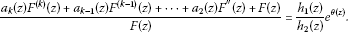(3.2)

If $\theta \left(z\right)$ is a constant, then Theorem 1.1 holds. Otherwise, $\theta \left(z\right)$ is a polynomial or a transcendental entire function, rewritten (3.2), we have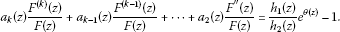(3.3)

Set , obviously, ${E}_{1}$ has finite linear measure. From Lemma 2.4, for any given $\epsilon >0$, there exists a set ${E}_{2}\subset \left(1,\mathrm{\infty }\right)$ that has finite logarithmic measure such that

$exp\left\{-{r}^{\alpha +\epsilon }\right\}\le |{a}_{j}\left(z\right)|\le exp\left\{{r}^{\alpha +\epsilon }\right\}\phantom{\rule{1em}{0ex}}\left(j=2,3,\dots ,k\right)$
(3.4)

holds for $|z|=r\notin \left[0,1\right]\cup {E}_{2}$, $r\to \mathrm{\infty }$, where $\alpha =max\left\{\sigma \left({a}_{j}\right)|j=2,\dots ,k\right\}<1$.

By Lemma 2.5, there exists a set ${E}_{3}\subset \left(1,\mathrm{\infty }\right)$ with finite logarithmic measure and a constant $B>0$ such that for all z satisfying $|z|=r\notin \left[0,1\right]\cup {E}_{3}$, we have

$|\frac{{F}^{\left(j\right)}\left(z\right)}{F\left(z\right)}|\le BT{\left(2r,F\right)}^{j+1}\phantom{\rule{1em}{0ex}}\left(j=2,\dots ,k\right).$
(3.5)

By (3.3)-(3.5), for $|z|=r\notin \left[0,1\right]\cup \left({E}_{1}\cup {E}_{2}\cup {E}_{3}\right)$, $r\to \mathrm{\infty }$, we have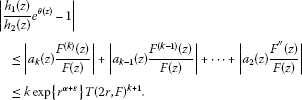Let $M\left(r,\frac{{h}_{1}\left(z\right)}{{h}_{2}\left(z\right)}{e}^{\theta \left(z\right)}-1\right)=|\frac{{h}_{1}\left(z\right)}{{h}_{2}\left(z\right)}{e}^{\theta \left(z\right)}-1|$, we obtain

$M\left(r,\frac{{h}_{1}\left(z\right)}{{h}_{2}\left(z\right)}{e}^{\theta \left(z\right)}-1\right)=|\frac{{h}_{1}\left(z\right)}{{h}_{2}\left(z\right)}{e}^{\theta \left(z\right)}-1|\le kexp\left\{{r}^{\alpha +\epsilon }\right\}T{\left(2r,F\right)}^{k+1}.$
(3.6)

From (3.6), we obtain

${\sigma }_{2}\left(f\right)={\sigma }_{2}\left(F\right)\ge \sigma \left(\frac{{h}_{1}\left(z\right)}{{h}_{2}\left(z\right)}{e}^{\theta \left(z\right)}-1\right)\ge 1.$
(3.7)

By Wiman-Valiron theory, there exists a set ${E}_{4}\subset \left(1,\mathrm{\infty }\right)$ having finite logarithmic measure, we choose z satisfying $|z|=r\notin \left[0,1\right]\cup {E}_{4}\cup {E}_{1}$, and $|F\left(z\right)|=|M\left(r,F\left(z\right)\right)|$, then

$\frac{{F}^{\left(j\right)}\left(z\right)}{F\left(z\right)}={\left(\frac{\upsilon \left(r\right)}{r}\right)}^{j}\left(1+o\left(1\right)\right)\phantom{\rule{1em}{0ex}}\left(j=2,\dots ,k\right),$
(3.8)

where $\upsilon \left(r\right)$ is the central index of $F\left(z\right)$. By (3.3), (3.5), (3.8), we obtain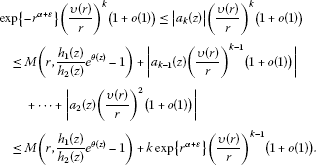Hence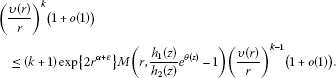(3.9)

By Lemma 2.6, (3.9), $\alpha <1$, and ${\sigma }_{2}\left(F\right)\ge 1$, we obtain

${\sigma }_{2}\left(f\right)={\sigma }_{2}\left(F\right)\le \sigma \left(\frac{{h}_{1}\left(z\right)}{{h}_{2}\left(z\right)}{e}^{\theta \left(z\right)}-1\right).$
(3.10)

By combining (3.7) and (3.10), we obtain

${\sigma }_{2}\left(f\right)={\sigma }_{2}\left(F\right)=\sigma \left(\frac{{h}_{1}\left(z\right)}{{h}_{2}\left(z\right)}{e}^{\theta \left(z\right)}-1\right).$
(3.11)

If $\theta \left(z\right)$ is polynomial, we know ${\sigma }_{2}\left(f\right)\in \mathbb{N}$; If $\theta \left(z\right)$ is transcendental, we get ${\sigma }_{2}\left(f\right)=\mathrm{\infty }$. Hence this contradicts the hypothesis of Theorem 1.1. Theorem 1.1 is thus proved.

## 4 Proof of Theorem 1.2

Under the hypothesis of Theorem 1.2, it is easy to get that

$\frac{{L}_{2}\left(f\right)-a}{f\left(z\right)-a}=h\left(z\right),$

where $h\left(z\right)$ is a meromorphic function. If $\sigma \left(h\right)<1$, by the proof of Theorem 1.1, similarly, we can prove

${\sigma }_{2}\left(f\right)=\sigma \left(h-{a}_{0}\right)\in \mathbb{N},$

which contradicts the fact that ${\sigma }_{2}\left(f\right)\notin \mathbb{N}$. This completes the proof of Theorem 1.2.

## 5 Proof of Theorem 1.3

Proof: By the Hadamard factorization theorem, we have

$\frac{A\left(f\right)-a\left(z\right)}{f\left(z\right)-a\left(z\right)}=B\left(z\right),$
(5.1)

where $B\left(z\right)$ is an entire function of order less than $\frac{1}{2}$. If $f\left(z\right)$ is polynomial, Theorem 1.3 holds. Next, we consider that $f\left(z\right)$ is transcendental. Set $F\left(z\right)=f\left(z\right)-a\left(z\right)$. Then $F\left(z\right)$ is transcendental, and $\sigma \left(F\right)<\frac{1}{2}$. Substituting $f\left(z\right)=F\left(z\right)+a\left(z\right)$ into (5.1), we have

$\frac{{a}_{k}\left(z\right){\mathrm{\Delta }}^{k}F\left(z\right)+{a}_{k-1}\left(z\right){\mathrm{\Delta }}^{k-1}F\left(z\right)+\cdots +{a}_{0}\left(z\right)F\left(z\right)+b\left(z\right)}{F\left(z\right)}=B\left(z\right),$
(5.2)

where $b\left(z\right)={a}_{k}\left(z\right){\mathrm{\Delta }}^{k}a\left(z\right)+\cdots +{a}_{1}\left(z\right)\mathrm{\Delta }a\left(z\right)+a\left(z\right)\left({a}_{0}\left(z\right)-1\right)$. By Lemma 2.1, there exists an ε-set ${E}_{n}$ such that

${\mathrm{\Delta }}^{j}F\left(z\right)\sim {F}^{\left(j\right)}\left(z\right)\phantom{\rule{1em}{0ex}}\left(j=1,2,\dots ,n\right),$
(5.3)

as $z\to \mathrm{\infty }$ in $\mathbb{C}\setminus {E}_{n}$. By Wiman-Valiron theory, there is a subset ${E}_{5}\subset \left(1,\mathrm{\infty }\right)$ with finite logarithmic measure. We choose z satisfying $|z|=r\notin {E}_{5}$ and $|F\left(z\right)|=|M\left(r,f\left(z\right)\right)|$, then we have

$\frac{{F}^{\left(j\right)}\left(z\right)}{F\left(z\right)}={\left(\frac{\upsilon \left(r\right)}{r}\right)}^{j}\left(1+o\left(1\right)\right)\phantom{\rule{1em}{0ex}}\left(j=1,2,\dots ,k\right),$
(5.4)

where $\upsilon \left(r\right)$ is the central index of $F\left(z\right)$. By (5.2)-(5.4), we have

${a}_{k}\left(z\right){\left(\frac{\upsilon \left(r\right)}{r}\right)}^{n}\left(1+o\left(1\right)\right)+\cdots +{a}_{1}\left(z\right)\left(\frac{\upsilon \left(r\right)}{r}\right)\left(1+o\left(1\right)\right)+\frac{b\left(z\right)}{F\left(z\right)}=B\left(z\right)-{a}_{0}\left(z\right).$
(5.5)

By Lemma 2.3, there exists a set ${E}_{6}\subset \left(1,\mathrm{\infty }\right)$ satisfying $\underline{log dens}\left({E}_{6}\right)=1$ such that

$\frac{M\left(r,b\left(z\right)\right)}{M\left(r,F\left(z\right)\right)}\to 0.$
(5.6)

Together with $\sigma \left(F\right)<1$, we get

${\left(\frac{\upsilon \left(r\right)}{r}\right)}^{n}\le {\left(\frac{\upsilon \left(r\right)}{r}\right)}^{n-1}\le \cdots \le \left(\frac{\upsilon \left(r\right)}{r}\right).$
(5.7)

By (5.5)-(5.7), we have

$|B\left(z\right)-{a}_{0}\left(z\right)|\le C\left(\frac{\upsilon \left(r\right)}{r}\right){r}^{C},$
(5.8)

where C is a constant. By Lemma 2.2, for any α satisfying $\mu <\alpha <\frac{1}{2}$, there exists a set ${E}_{7}$ with $\underline{log dens}\left({E}_{7}\right)\ge 1-\frac{\mu }{\alpha }$, such that

$|B\left(r{e}^{i\theta }\right)-{a}_{0}\left(r{e}^{i\theta }\right)|\ge M\left(r,{\left(B-{a}_{0}\right)}^{\lambda }\right),$
(5.9)

for $|z|=r\in {E}_{7}$, where $\lambda =cos\pi \alpha$.

Note the characteristic function of ${E}_{6}$ and ${E}_{7}$ such that the relation

${\chi }_{{E}_{6}\cap {E}_{7}\left(t\right)}={\chi }_{{E}_{6}}\left(t\right)+{\chi }_{{E}_{7}}\left(t\right)-{\chi }_{{E}_{6}\cup {E}_{7}}\left(t\right).$

Obviously, $\overline{log dens}\left({E}_{6}\cup {E}_{7}\right)\le 1$. Hence we obtain

$1-\frac{\mu }{\alpha }\le \overline{log dens}\left({E}_{6}\right)+\underline{log dens}\left({E}_{7}\right)-\overline{log dens}\left({E}_{6}\cup {E}_{7}\right)\le \overline{log dens}\left({E}_{6}\cap {E}_{7}\right).$

Thus, the upper logarithmic density of $\left({E}_{6}\cap {E}_{7}\right)\setminus \left({E}_{n}\cup {E}_{4}\cup {E}_{5}\cup \left[0,1\right]\right)$ is also more than $1-\frac{\mu }{\alpha }$. By (5.8) and (5.9), for $z\in \left({E}_{6}\cap {E}_{7}\right)\setminus \left({E}_{n}\cup {E}_{4}\cup {E}_{5}\cup \left[0,1\right]\right)$, we have

$M\left(r,{\left(B-{a}_{0}\right)}^{\lambda }\right)\le C\left(\frac{\upsilon \left(r\right)}{r}\right){r}^{C}.$

If $B\left(z\right)$ is transcendental, we get $\sigma \left(f\right)=\mathrm{\infty }$, which contradicts our assumption that $\sigma \left(f\right)<\frac{1}{2}$. So $B\left(z\right)$ is polynomial. This proves Theorem 1.3.

## References

1. Hayman W: Meromorphic Functions. Clarendon Press, Oxford; 1964.

2. Laine I: Nevanlinna Theory and Complex Differential Equations. Walter de Gruyter, Berlin; 1993.

3. Yang CC, Yi HX: Uniqueness of Meromorphic Functions. Kluwer Academic, Dordrecht; 2003.

4. Whittaker JM Cambridge Tracts in Math. Phys 33. In Interpolatory Function Theory. Cambridge University Press, Cambridge; 1935.

5. Brück R: On entire functions which share one value CM with their first derivatives. Results Math. 1996, 30: 21–24.

6. Gundersen GG, Yang LZ: Entire functions that share one value with one or two of their derivatives. J. Math. Anal. Appl. 1998, 223: 88–95. 10.1006/jmaa.1998.5959

7. Yang LZ: Solution of a differential equation and its application. Kodai Math. J. 1999, 22: 458–464. 10.2996/kmj/1138044097

8. Chen ZX, Shon K: On conjecture of R. Brück concerning the entire function sharing one value CM with its derivative. Taiwan. J. Math. 2004, 8: 235–244.

9. Wang J: Uniqueness of entire function sharing a small function with its derivative. J. Math. Anal. Appl. 2010, 362: 387–392. 10.1016/j.jmaa.2009.09.052

10. Hayman W: The local growth of power series: a survey of the Wiman-Valiron method. Can. Math. Bull. 1974, 17: 317–358. 10.4153/CMB-1974-064-0

11. Bergweiler W, Langley JK: Zeros of differences of meromorphic functions. Math. Proc. Camb. Philos. Soc. 2007, 142: 133–147. 10.1017/S0305004106009777

12. Barry PD: On a theorem of Besicovitch. Q. J. Math. 1963, 14: 293–302. 10.1093/qmath/14.1.293

13. Chen ZX: The zero, pole and order meromorphic solutions of differential equations with meromorphic coefficients. Kodai Math. J. 1996, 19: 341–354. 10.2996/kmj/1138043651

14. Gundersen G: Estimates for the logarithmic derivative of a meromorphic function, plus similar estimates. J. Lond. Math. Soc. 1988, 37: 88–104.

15. Chen ZX, Yang CC: Some furthers on the zeros and growths of entire solutions of second order linear differential equations. Kodai Math. J. 1999, 22: 273–285. 10.2996/kmj/1138044047

## Acknowledgements

The work was supported by the NNSF of China (No. 10771121), the NSF of Shangdong Province, China (No. Z2008A01) and Shandong university graduate student independent innovation fund (yzc11024).

## Author information

Authors

### Corresponding author

Correspondence to Yin Hong Cao.

### Competing interests

The authors declare that they have no competing interests.

### Authors’ contributions

YL and YH completed the main parts of the paper. YL, YH and HX corrected the main theorems. All authors read and approved the final manuscript.

## Rights and permissions

Reprints and Permissions

Liu, Y., Cao, Y.H. & Yi, H.X. Some results of certain types of difference and differential equations. Adv Differ Equ 2012, 127 (2012). https://doi.org/10.1186/1687-1847-2012-127

• Accepted:

• Published:

• DOI: https://doi.org/10.1186/1687-1847-2012-127

### Keywords

• entire functions
• uniqueness
• complex difference
• share value